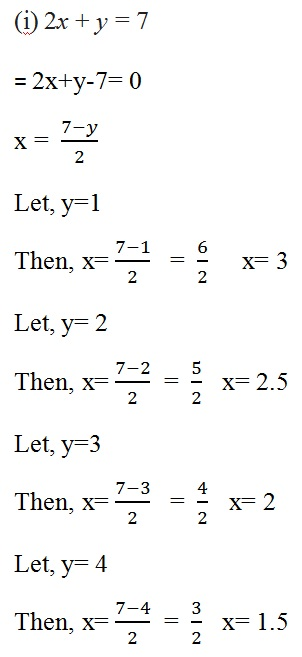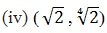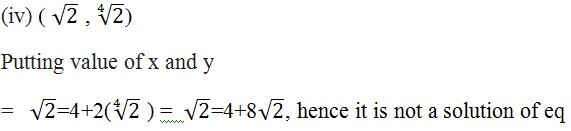Study Materials: ncert solutions

Our ncert solutions for Exercise 4.2 Class 9 maths 4. Linear Equation In Two Variables - ncert solutions - Toppers Study is the best material for English Medium students cbse board and other state boards students.

Solutions ⇒ Class 9th ⇒ Mathematics ⇒ 4. Linear Equation In Two Variables

# Exercise 4.2 Class 9 maths 4. Linear Equation In Two Variables - ncert solutions - Toppers Study

Topper Study classes prepares ncert solutions on practical base problems and comes out with the best result that helps the students and teachers as well as tutors and so many ecademic coaching classes that they need in practical life. Our ncert solutions for Exercise 4.2 Class 9 maths 4. Linear Equation In Two Variables - ncert solutions - Toppers Study is the best material for English Medium students cbse board and other state boards students.

## Exercise 4.2 Class 9 maths 4. Linear Equation In Two Variables - ncert solutions - Toppers Study

CBSE board students who preparing for class 9 ncert solutions maths and Mathematics solved exercise chapter 4. Linear Equation In Two Variables available and this helps in upcoming exams 2023-2024.

### You can Find Mathematics solution Class 9 Chapter 4. Linear Equation In Two Variables

• All Chapter review quick revision notes for chapter 4. Linear Equation In Two Variables Class 9
• NCERT Solutions And Textual questions Answers Class 9 Mathematics
• Extra NCERT Book questions Answers Class 9 Mathematics
• Importatnt key points with additional Assignment and questions bank solved.

NCERT Solutions do not only help you to cover your syllabus but also will give to textual support in exams 2023-2024 to complete exercise 4.2 maths class 9 chapter 4 in english medium. So revise and practice these all cbse study materials like class 9 maths chapter 4.2 in english ncert book. Also ensure to repractice all syllabus within time or before board exams for ncert class 9 maths ex 4.2 in english.

See all solutions for class 9 maths chapter 4 exercise 4 in english medium solved questions with answers.

### Exercise 4.2 class 9 Mathematics Chapter 4. Linear Equation In Two Variables

• Exercise 4.2 Class 9 Maths 4. Linear Equation In Two Variables - Ncert Solutions - Toppers Study
• Class 9 Ncert Solutions
• Solution Chapter 4. Linear Equation In Two Variables Class 9
• Solutions Class 9
• Chapter 4. Linear Equation In Two Variables Exercise 4.2 Class 9

## Exercise 4.2 Class 9 maths 4. Linear Equation In Two Variables - ncert solutions - Toppers Study

Chapter 4. Linear Equation In Two Variables

Exercise 4.2

1. Which one of the following options is true, and why?

y = 3x + 5 has

(i) a unique solution,  (ii) only two solutions,

Solution

(iii) infinitely many solution, because we can put many value of x and can get many solution of y.

2. Write four solutions for each of the following equations:

(i) 2x + y = 7

(ii) πx + y = 9

(iii) x = 4y

Solution3. Check which of the following are solutions of the equation – 2= 4 and which are not:

(i) (0, 2)

(ii) (2, 0)

(iii) (4, 0)Solution :

x = 4 + 2y

(i) (0,2)

Putting value of x and y

=  0 = 4 + 2(2)

=  0 = 4 + 4    =  0 = 8, hence it is not a solution of eq

(ii) (2,0)

Putting value of x and y

=  2 = 4 + 2(0)   =  2 = 4, hence it is also not a solution of eq

(iii) (4,0)

Putting value of x and y

=   4 = 4 + 2(0)    =  4 = 4, hence it is a solution of eq(v) (1,1)

Putting value of x and y

=   1 = 4 + 2(1)   = 1= 6, hence, it is not a solution of eq

4. Find the value of k, if = 2, = 1 is a solution of the equation 2+ 3k.

Solution :

2x + 3y = k

Putting the value of x and y

2(2) + 3(1) = k   = 4+3 = k    = k = 7

##### Other Pages of this Chapter: 4. Linear Equation In Two Variables

Important Study materials for classes 06, 07, 08,09,10, 11 and 12. Like CBSE Notes, Notes for Science, Notes for maths, Notes for Social Science, Notes for Accountancy, Notes for Economics, Notes for political Science, Noes for History, Notes For Bussiness Study, Physical Educations, Sample Papers, Test Papers, Mock Test Papers, Support Materials and Books.Mathematics Class - 11th

NCERT Maths book for CBSE Students.

books

## Study Materials List:

##### Solutions ⇒ Class 9th ⇒ Mathematics
1. Number Systems
2. Polynomials
3. Coordinate Geometry
4. Linear Equation In Two Variables
5. Introduction To Euclid’s Geometry
6. Lines and Angles
7. Triangles